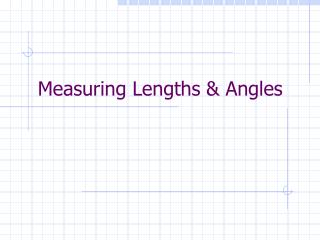DownloadDownload PresentationMeasuring Lengths & Angles

# Measuring Lengths & Angles

Download Presentation## Measuring Lengths & Angles

- - - - - - - - - - - - - - - - - - - - - - - - - - - E N D - - - - - - - - - - - - - - - - - - - - - - - - - - -
##### Presentation Transcript

1. Measuring Lengths & Angles

2. Measure of Segment AB The length of AB is the absolute value of a-b. |a-b| or |b-a| A B a b

3. Congruence • Objects that are congruent have the same measure

4. Segment Addition Postulate If point R is between points P and Q, then RP + PQ = PQ P R Q

5. Angle Measure (using a protractor) • Line up site with vertex • Line up one side with 0° • Read angle measure using other side

6. Angle Addition Postulate If point S is in the interior of <PQR, then m<PQS + m<SQR = m<PQR P S Q R

7. Special Angle Sums If the sum of the measures of two angle = • 90° => Complementary • 180° => Supplementary